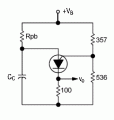# Substituting a 2n6028 for a 2n4891 unijunction transistor

#### johnnyinwa

Joined Jun 24, 2013
52
Heh guys -- new user here,
Well I am working on an electronic organ project from Forrest M. Mimms the III book "Getting Started in Electronics" from Radio Shack page 108. The circuit calls for a 2n4891 unijunction transistor which is now obsolete and very expensive. I know I can substitute a 2n6028 PUT that will do the job but I need to know the values of the programming resistors to make the substitution work. Please explain in depth as I am new to the field of electronics. An attached schematic would be nice. Thank you so much for your help with this issue!

johnnyinwa.#### Attachments

• 421 KB Views: 106

#### crutschow

Joined Mar 14, 2008
27,954
Below is the basic circuit using the 2N6028 PUT which should have characteristics reasonably close to the 2N4891. Rpb is the timing resistor selected by the switch.

One difference is that the PUT has a lower ratio between the peak and valley currents so it can not operate with as wide a range of Rpb resistors as the 2N4891*. If you have a problem with it not oscillating for certain resistor values you may have to tweak the values of the resistors and consequently the value of the timing capacitor Cc in proportion.

*Note: Rpb has to be small enough so it exceeds the Ip value when the capacitor voltage reaches about 60% of the supply voltage and large enough so the current falls below the Iv value at the end of the capacitor discharge. For the two resistor values shown, Ip is $$\leq$$50μA and Iv is $$\geq$$1mA.

Good luck.#### absf

Joined Dec 29, 2010
1,952
The only UJT I know was the 2N2646 which I have a few. They were given to me by my friend.

I found a good section from the Practical Electronics for Inventers describing the UJT and PUT as attached...

Allen

#### Attachments

• 463.8 KB Views: 182

#### johnnyinwa

Joined Jun 24, 2013
52
Thanks for the replies,
Please look at the schematic on the original post and tell me if there is a better substitute for the 2n4891. I was thinking a 555 might do the trick but I don't know how to hook it up? What is the output waveform for the 2n4891? By the way I am using a 9 volt supply for the circuit. Another question -- why is the 10k resistor "R10" needed in the circuit? As far as the coupling capacitor c2 goes is there a standard value to use or can it be calculated? Thank you so much for your help here guys.#### Attachments

• 421 KB Views: 59

#### crutschow

Joined Mar 14, 2008
27,954
A 555 connected as an astable multivibrator may work for you. You would vary R1 to change the frequency. But note that the output is more of a square-wave then a pulse.

You can make that into a short pulse, similar to the unijunction's output, by running the signal through C2 with the value of C2 reduced to give the pulse width desired. You also need to add a diode to ground at C2's output (anode to ground) to suppress the reverse voltage pulse from the square-wave's negative slope.

The output of a unijunction is a short pulse.

R10 is needed to provide DC current for the base of Q2. The C2 capacitor can not pass this DC.

The value of C2 is somewhat arbitrary. It just has to be large enough to pass the pulse from the unijunction with sufficient current to turn on Q2. Likely it could be larger or smaller without significant change in the sound.

•johnnyinwa

#### johnnyinwa

Joined Jun 24, 2013
52
I still don't understand why r10 in the attached schematic is needed. I understand that its dumping a small amount of dc current to the base of q2 but why does q2 need the current. Isn't the dc current just noise that gets in the way of the signal from q1?#### Attachments

• 421 KB Views: 47

#### crutschow

Joined Mar 14, 2008
27,954
I still don't understand why r10 in the attached schematic is needed. I understand that its dumping a small amount of dc current to the base of q2 but why does q2 need the current. Isn't the dc current just noise that gets in the way of the signal from q1?The pulses go from Q1 through C2 to the base of Q2. These pulses generate a short positive pulse of current through the base of Q2. Since the base-emitter junction looks like a diode, there is no reverse current to replace the capacitor charge lost with each current pulse. Eventually the capacitor output will sit at a negative voltage below the peak value of the positive pulses so Q2 no longer turns on. R10 provides a small current to replace this charge lost.

If the pulse value from Q1 was high enough then you could eliminate C2 and R10 since now there is no capacitor charge that needs replacing.

•johnnyinwa